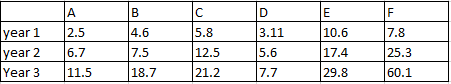### CAT 1996 Question 1

Instructions

Direction for questions: Answer the questions based on the following information. The data given in the table shows the investment details in country ‘Fortune Land’ of companies A, B, C, D, E and F. Figures in the table are in US dollars in billions.Question 1

# What is the percentage increase in investment of B, C, D and E from year 1 to year 3?

Solution

Total investment by B, C, D and E in year 1 = 24.11 Billion

Total investment by B, C, D and E in year 3 = 77.4 Billion

Increase = 77.4 - 24.11 = 53.29 Billion

% Increase = $$\frac{53.29}{24.11}*100$$ = 221%